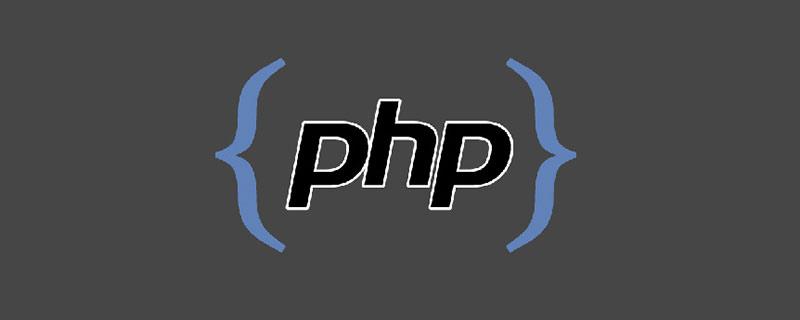# 让PHP能够调用C的函数：FFI扩展（附代码）# 让PHP能够调用C的函数-FFI扩展

## 什么是 FFI

FFI ， Foreign Function Interface，外部函数接口。这个扩展允许我们加载一些公共库（.dll、.so），其实也就是可以调用一些 C 的数据结构及函数。它已经是随 PHP 源码发布的一个扩展了，在编译的时候可以加上 --with-ffi 来直接编译到 PHP 程序中。

```cd php-7.4.4/ext/ffi/
phpize
./configure
make && make install```

## 使用 FFI 调用 C 的函数

```// 创建一个 FFI 对象，加载 libc 并且导入 printf 函数
\$ffi_printf = FFI::cdef(
"int printf(const char *format, ...);", // C 的定义规则
"libc.so.6"); // 指定 libc 库
// 调用 C 的 printf 函数
\$ffi_printf->printf("Hello %s!\n", "world"); // Hello World

// 加载 math 并且导入 pow 函数
\$ffi_pow = FFI::cdef(
"double pow(double x, double y);",
"libboost_math_c99.so.1.66.0");
// 这里调用的是 C 的 pow 函数，不是 PHP 自己的
echo \$ffi_pow->pow(2,3), PHP_EOL; // 8```

## 定义变量和数组

```// 创建一个 int 变量
\$x = FFI::new("int");
var_dump(\$x->cdata); // int(0)

// 为变量赋值
\$x->cdata = 5;
var_dump(\$x->cdata); // int(5)

// 计算变量
\$x->cdata += 2;
var_dump(\$x->cdata); // int(7)

// 结合上面的两个 FFI 对象操作

echo "pow value:", \$ffi_pow->pow(\$x->cdata, 3), PHP_EOL;
// pow value:343
\$ffi_printf->printf("Int Pow value is : %f\n", \$ffi_pow->pow(\$x->cdata, 3));
// Int Pow value is : 343.000000

// 创建一个数组
\$a = FFI::new("long");
// 为数组赋值
for (\$i = 0; \$i < count(\$a); \$i++) {
\$a[\$i] = \$i;
}
var_dump(\$a); // int(25)

\$sum = 0;
foreach (\$a as \$n) {
\$sum += \$n;
}
var_dump(\$sum); // int(523776)

var_dump(count(\$a)); // int(1024) 数组长度
var_dump(FFI::sizeof(\$a)); // int(8192)，内存大小```

## 总结

`https://github.com/zhangyue0503/dev-blog/blob/master/php/202004/source/%E8%AE%A9PHP%E8%83%BD%E5%A4%9F%E8%B0%83%E7%94%A8C%E7%9A%84%E5%87%BD%E6%95%B0-FFI%E6%89%A9%E5%B1%95.php`### 全部评论我要评论

• 取消发布评论发送
• 1/1